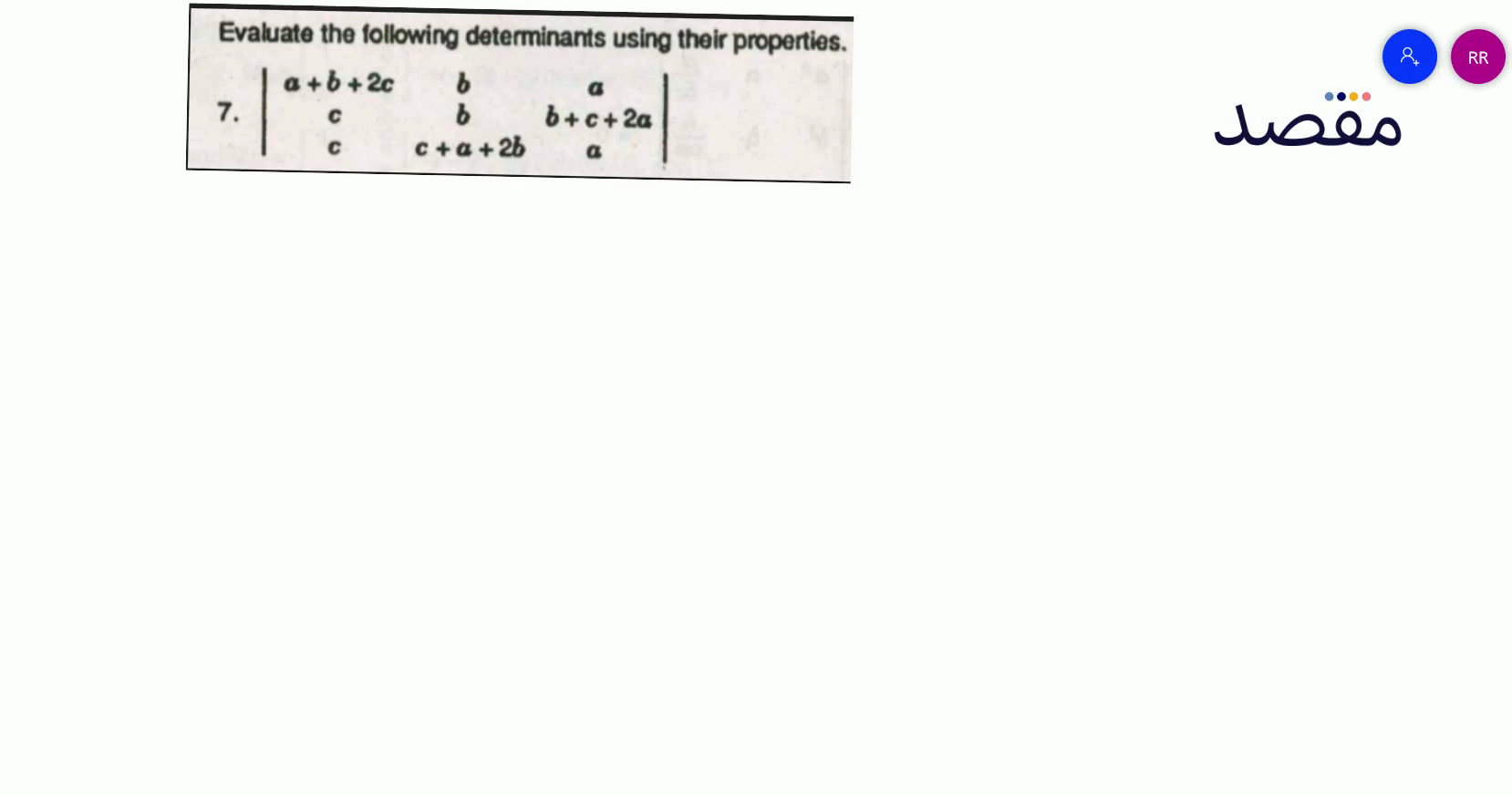# Classes

Change the way you learn with Maqsad's classes. Local examples, engaging animations, and instant video solutions keep you on your toes and make learning fun like never before!

Class 9Class 10First YearSecond Year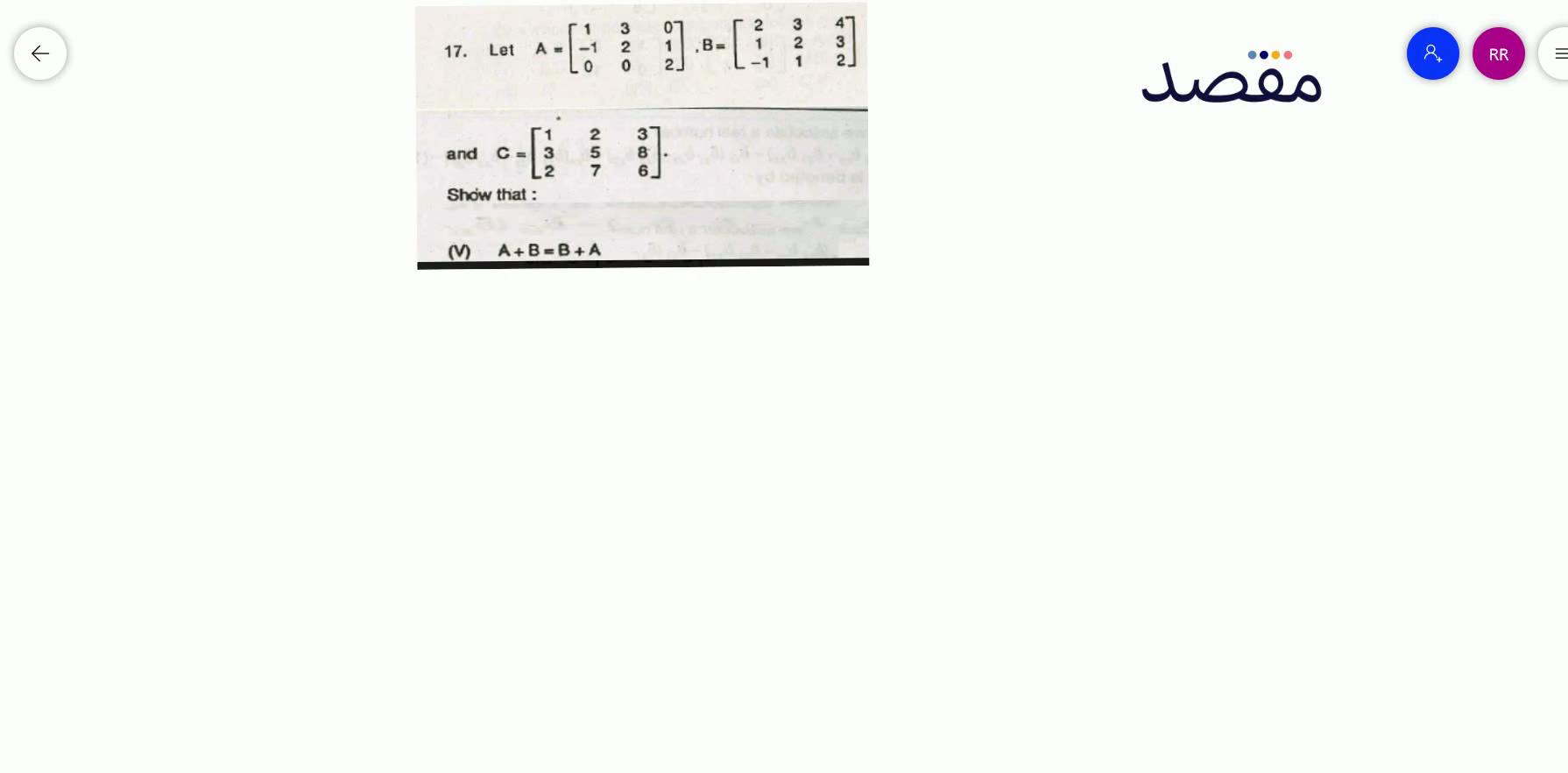### 17. Let \mathrm{A}=\left[\begin{array}{ccc}1 & 3 & 0 \\ -1 & 2 & 1 \\ 0 & 0 & 2\end{array}\right] \mathrm{B}=\left[\begin{array}{ccc}2 & 3 & 4 \\ 1 & 2 & 3 \\ -1 & 1 & 2\end{array}\right] and C=\left[\begin{array}{lll}1 & 2 & 3 \\ 3 & 5 & 8 \\ 2 & 7 & 6\end{array}\right] Show that :(V) \mathrm{A}+\mathrm{B}=\mathrm{B}+\mathrm{A}^{-7}=\pm=### Evaluate the following determinants using their properties.8. \left|\begin{array}{lll}x & y & z \\ z & x & y \\ y & z & x\end{array}\right|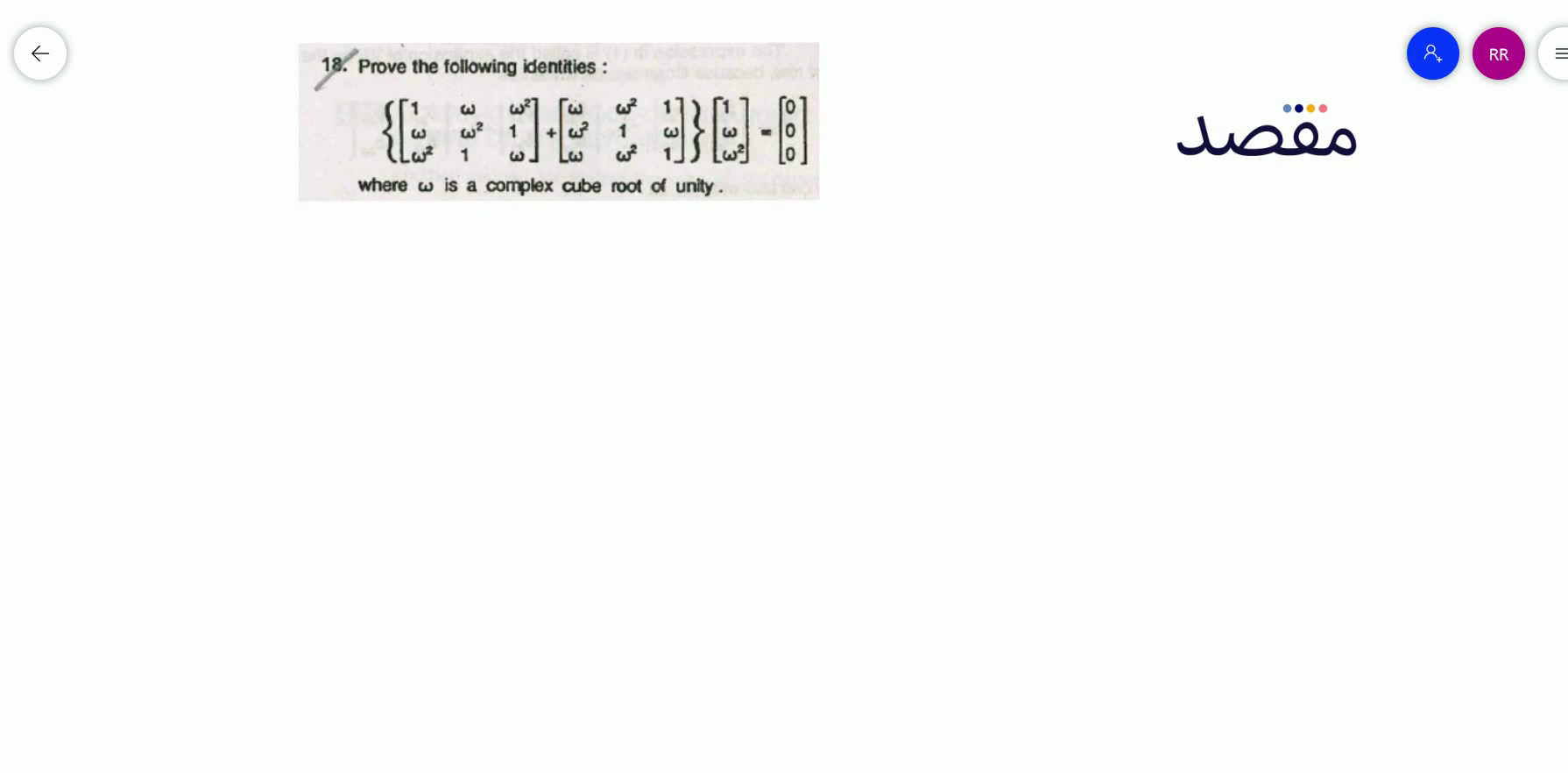### 18. Prove the following identities :$\left\{\left[\begin{array}{lll}1 & \omega & \omega^{2} \\\omega & \omega^{2} & 1 \\\omega^{2} & 1 & \omega\end{array}\right]+\left[\begin{array}{lll}\omega & \omega^{2} & 1 \\\omega^{2} & 1 & \omega \\\omega & \omega^{2} & 1\end{array}\right]\right\}\left[\begin{array}{l}1 \\\omega \\\omega^{2}\end{array}\right]=\left[\begin{array}{l}0 \\0 \\0\end{array}\right]$where \omega is a complex cube root of unity.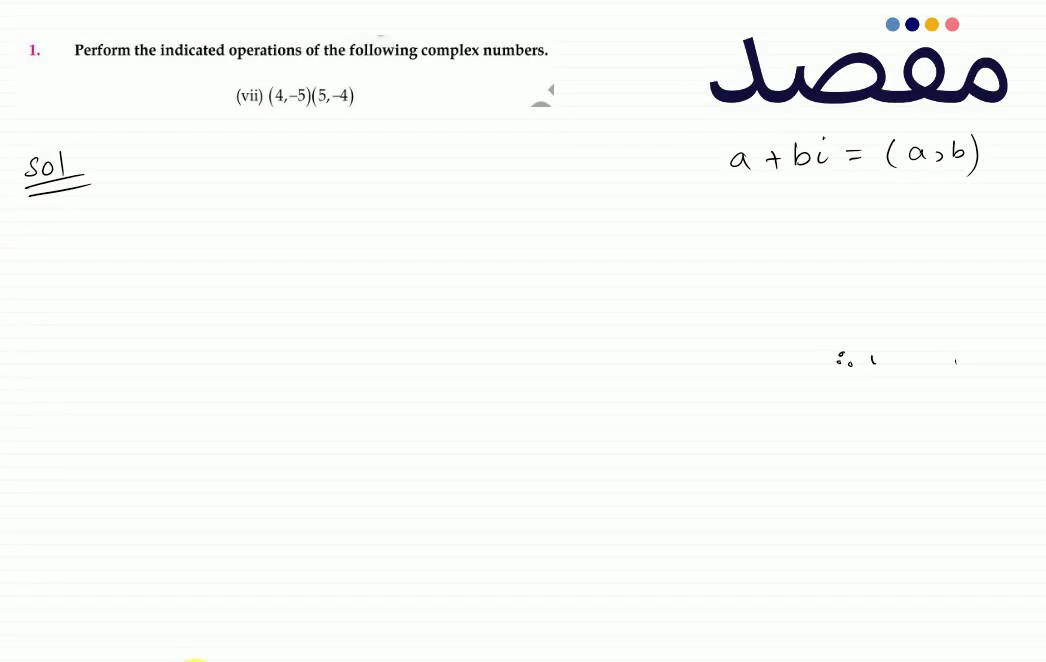### 1. Use matrices if possible to solve the following systems of linear equations by:(i) the matrix inversion method (ii) the Cramers rule.(vii)$\begin{array}{l}2 x-2 y=4 \\-5 x-2 y=-10\end{array}$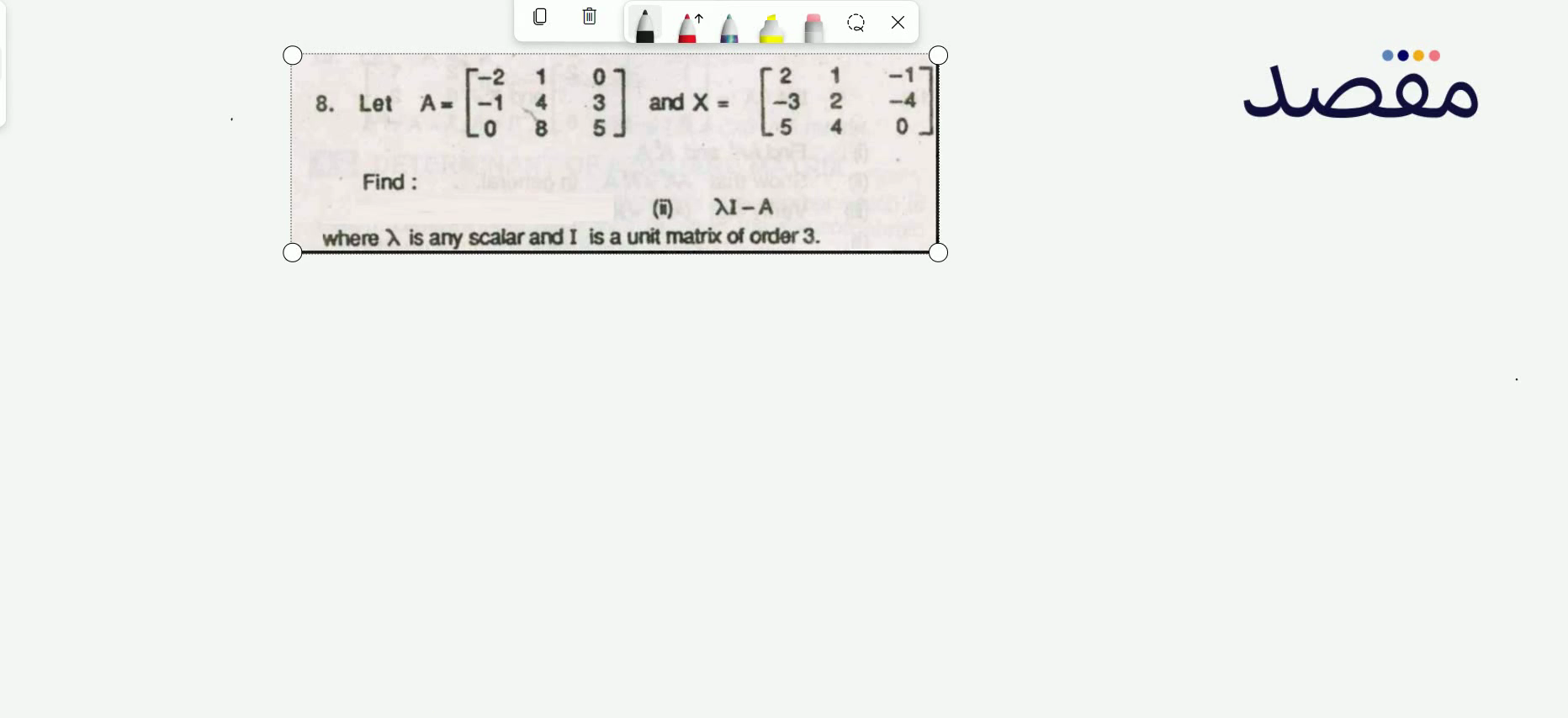### 8. Let A=\left[\begin{array}{ccc}-2 & 1 & 0 \\ -1 & 4 & 3 \\ 0 & 8 & 5\end{array}\right] and X=\left[\begin{array}{ccc}2 & 1 & -1 \\ -3 & 2 & -4 \\ 5 & 4 & 0\end{array}\right] Find:(ii) \lambda I-A where \lambda is any scalar and I is a unit matrix of order 3 .